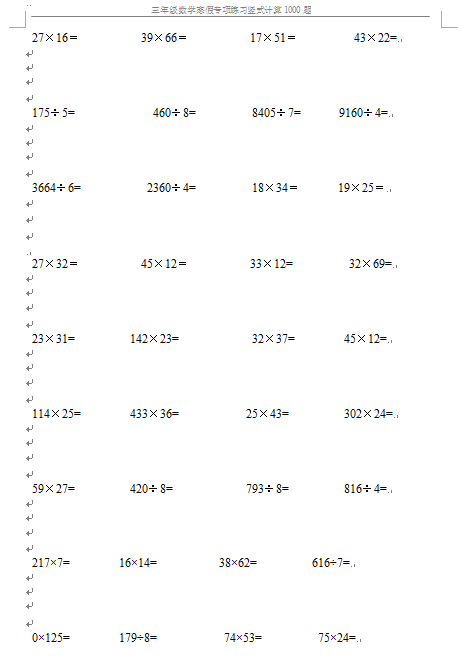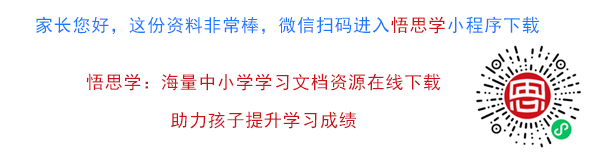27×16＝           39×66＝           17×51＝          43×22=

175÷5=                            460÷8=                    8405÷7=              9160÷4=

3664÷6=                        2360÷4=                    18×34＝       19×25＝

27×32＝           45×12＝           33×12=                    32×69=

### 【WORD打印文档33页】小学三年级下册数学寒假专项练习竖式计算1000题电子打印版资料下载##### 评论信息